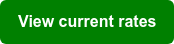# Loan-to-Value | How to calculate LTV for a mortgage

Jim Quist Jan 3, 2023 12:05:50 PM### Contents

Loan-to-value (LTV) ratio is a measure that compares the amount of the mortgage loan to the value of the property. Lenders use it to determine the risk of lending you money for a mortgage.

To calculate the LTV ratio for your home purchase, divide the loan amount by the property's value. For example, if you want to buy a property valued at \$300,000 and your home loan is \$240,000, the LTV ratio is 80%.

#### Example - Calculate 80% LTV

Here is an example of how to calculate the loan-to-value (LTV) ratio for a mortgage:

Let's say that you want to buy a house for \$300,000. You've saved up \$60,000 for a down payment and will need a mortgage for the remaining \$240,000.

To calculate the LTV ratio, divide the loan amount by the value of the property:

• LTV ratio = \$240,000 / \$300,000 = 0.80

The LTV ratio in this example is 80%. This means that the loan represents 80% of the property's value.

#### Example - Calculate 97% LTV

Here is an example of how to calculate the loan-to-value (LTV) ratio for a mortgage when the purchase price is \$500,000, and the LTV is 97%:

If the property's purchase price is \$500,000 and the LTV is 97%, you borrow 97%, or \$485,000. The remaining 3%, or \$15,000, will come from your down payment.

To calculate the LTV ratio, divide the loan amount by the value of the property:

• LTV ratio = \$485,000 / \$500,000 = 0.97

The LTV ratio in this example is 97%. This means that the loan represents 97% of the property's value.

Lenders generally prefer to see a lower LTV ratio, as it indicates that you have a more significant equity stake in the property and are, therefore, less likely to default on the loan. Lenders may charge a higher interest rate for a loan with a higher LTV ratio.## Do LTV limits differ depending on the loan type?

The loan-to-value (LTV) ratio limits for mortgage loans can vary depending on the type of mortgage and the lender's requirements.

For a conventional mortgage, the LTV ratio limits can vary depending on the lender and other factors, such as your credit score, the type of property you are financing, and whether or not you'll live in the property. For example, some lenders may require a lower LTV ratio for a conventional mortgage. In comparison, others may be willing to consider a higher LTV ratio on a case-by-case basis.

For example, the maximum LTV for a conventional loan is 97% if you're buying a single-family home and you will live there. This means your down payment must be 3% or more.

On the other hand, the maximum LTV would be 85% if you're buying a 2-unit property and you'll live in one of the units and rent out the other.

For FHA loans, the LTV ratio limits are generally higher than those for conventional mortgages. For example, lenders may allow an LTV ratio of up to 96.5% for an FHA loan, for a single-family home, or a 2-to-4-unit property, although this can vary depending on the lender and other factors.

VA loans may also have higher LTV ratio limits than conventional mortgages. For example, lenders may allow an LTV ratio of up to 100% for a VA loan, meaning that a veteran eligible for a VA loan may not need a down payment when buying a home.

LTV ratio limits for mortgage loans are not set in stone and can vary depending on your circumstances. Therefore, it's always a good idea to check with a lender like NewCastle Home Loans for specific LTV ratio requirements.

To see how much you need for a down payment, check out our Mortgage Calculator. Then, feel confident in buying a home because you know what to expect.